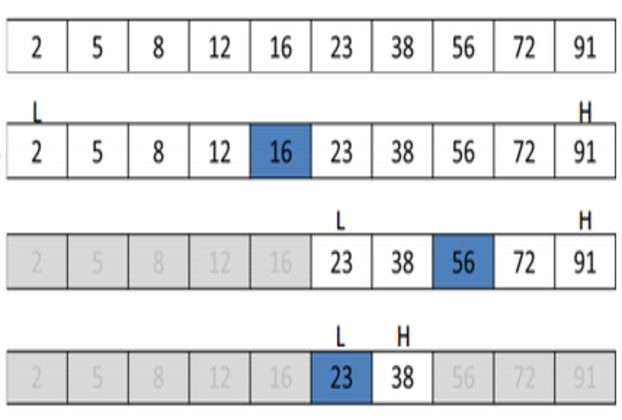# C# Technique: Simple Binary Search Example

Binary Search is the most simple, straight-forward but efficient manner of finding a key from a sorted array. It is carried out by repeatedly dividing the search array in half. If the value of the search key is less than the item in the middle of the half in focus, it narrows down the interval to the lower half. Otherwise narrows it to the upper half. This is done recursively until the required key element is found. Binary search algorithm is far more efficient because it does not have to search for the required key in a sequential order, hence many a times only a handful of recursive lookups pull in the result.

Here’s a simple C# example which figures out the index of the key 12 in a sorted array. Note that the function binarySearch() calls itself recursively by dividing the source array into two halves until it successfully finds the key. Then it returns the index of the key in the array.

``````        static int binarySearch(int[] arr, int low, int high, int key)
{
if (high < low)
return -1;

int mid = (low + high) / 2;

if (key == arr[mid])
return mid;

if (key > arr[mid])
return binarySearch(arr, (mid + 1), high, key);

return binarySearch(arr, low, (mid - 1), key);
}

static void Main(string[] args)
{
int[] arr = { 1, 2, 3, 5, 6, 7, 8, 9, 10, 12, 14, 15 };
int n = arr.Length-1;
int key = 12;

int result = binarySearch(arr, 0, n, key);
}``````